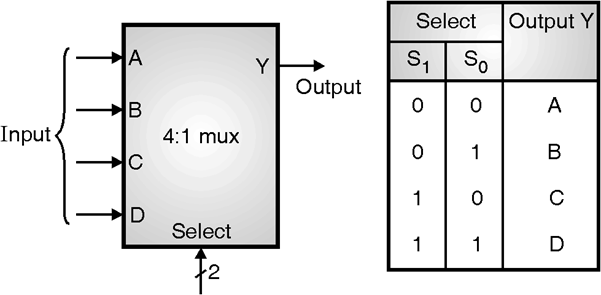Home > VHDL > Logic Circuits > 4 1 Mux

Implementation of 4:1 Mux :

Multiplexers can be modeled in various ways. Figure below shows the details of 4:1 multiplexor. The four common methods are to:

1) Using if -- elseif statements.

2) Using case statement.

3) Conditional signal assignment.

4) Selected signal assignment1) 4:1 mux using if statement :

An If statement generally produces priority-encoded logic, therefore it results in slower circuit overall. An If statement can contain a set of different expressions. Most current synthesis tools can determine if the if-elsif conditions are mutually exclusive, and will not create extra logic to build the priority tree. The following examples use an If construct in a 4:1 multiplexer design.

library ieee;

use ieee.std_logic_1164.all;

entity MUX4_1 is

port (Sel : in std_logic_vector(1 downto 0);

A, B, C, D : in std_logic;

Y : out std_logic );

end MUX4_1;

architecture behavior1 of MUX4_1 is

begin

process (Sel, A, B, C, D) -- Using an if statement is not as clear as using case

begin

if (Sel = "00") then Y<= A;

elsif (Sel = "01") then Y<= B;

elsif (Sel = "10") then Y<= C;

else Y<= D;

end if ;

end process ;

end behavior1;

2) Multiplexer using case statement :

The following examples shows 4:1 Multiplexer using a case statement.

library ieee;

use ieee.std_logic_1164.all;

entity MUX4_1 is

port (Sel : in std_logic_vector(1 downto 0);

A, B, C, D : in std_logic;

Y : out std_logic );

end MUX4_1;

architecture behavior2 of MUX4_1 is

begin

process (Sel, A, B, C, D)

begin

case Sel is

when "00" => Y<=A;

when "01" => Y<=B;

when "10" => Y<=C;

when "11" => Y<=D;

when others => Y<=A;

end case ;

end process ;

end behavior2;

3) Using conditional signal assignment :

library ieee;

use ieee.std_logic_1164.all;

entity MUX4_1 is

port (Sel : in std_logic_vector(1 downto 0);

A, B, C, D : in std_logic;

Y : out std_logic );

end MUX4_1;

architecture behavior3 of MUX4_1 is

begin

Y <= A when Sel = "00" else

B when Sel = "01" else

C when Sel = "10" else

D; - - when Sel = "11";

end behavior3;

4) Using selected signal assignment :

library ieee;

use ieee.std_logic_1164.all;

entity MUX4_1 is

port (Sel : in std_logic_vector(1 downto 0);

A, B, C, D : in std_logic;

Y : out std_logic );

end MUX4_1;

architecture behavior4 of MUX4_1 is

begin

with Sel select

Y<= A when "00",

B when "01",

C when "10",

D when "11",

A when others;

end behavior4;